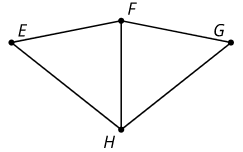# G.2.5 In Class Practice

Write a sequence of rigid motions to take figure ABC to figure DEF.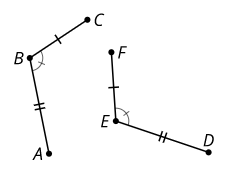Prove the circle centered at A is congruent to the circle centered at C.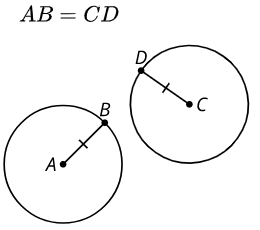Which conjecture is possible to prove?

Select all that apply
• A
• B
• C
• D

Match each statement using only the information shown in the pairs of congruent triangles. A. The 2 sides and the included angle of one triangle are congruent to 2 sides and the included angle of another triangle. B. The 2 angles and the included side of one triangle are congruent to 2 angles and the included side of another triangle. C. In the 2 triangles there are 3 pairs of congruent sides. 1. 2. 3.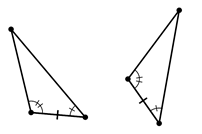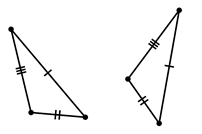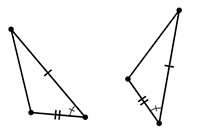Triangle HEF is the image of triangle HGF after a reflection across line FH. Write a congruence statement for the 2 congruent triangles.